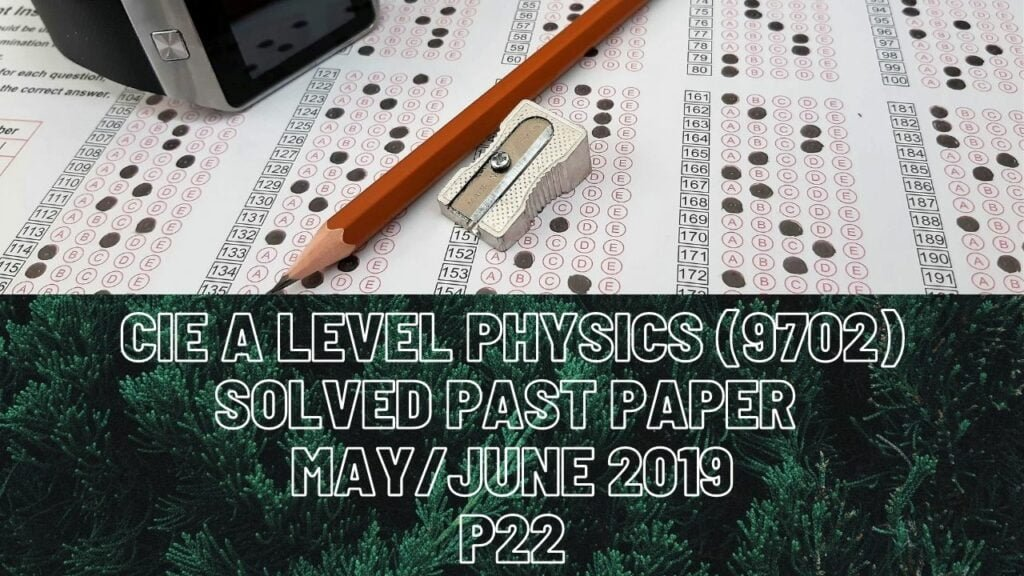A Level Physics### CIE A Level Physics (9702) Solved Past Paper May/June 2019 P22

1a) To convert % uncertainty to absolute uncertainty, divide % uncertainty by 100 and multiply that to the value for which you are calculating absolute uncertainty for.

b ii) Since all the quantities are either getting multiplied or divided in the formula for pressure, we must first add % uncertainties of all individual quantities then divide it by 100 and multiply that with value of pressure or whichever value you are finding absolute uncertainty for. Keep in mind, your final % or absolute uncertainty should be corrected to appropriate number of significant figures like in this Q, previous two uncertainties (2.8% & 1.6%) were corrected to 2 significant figures so your answer needs to be corrected to 2 significant figures too.

2a) DON’T write force is directly proportional to mass or acceleration. Although the statement is write, you are expected to come up with an answer in terms of momentum. F=m*a where a=(v-u)/t and this becomes rate of change of momentum.

bii) 1. Acceleration from t=0s until t=12.5s is constant so you can calculate your gradient which gives acceleration by taking any 2 co-ordinates within this time interval for this Q.

3. For this Q, you are given with two forces already and the resultant force. The car is moving to the right thus the frictional force of magnitude of 510N acts to the left of car. Also, tension in tow-bar is acting towards the left of car because that acts against the pulling force (driving force in this Q). A force should be exerted by car’s engine which makes it possible for the car to accelerate to the right side, this means the force acting towards the right is much greater than the sum of both the forces acting to the left of car. When you subtract the sum of tension and frictional force from engine’s force, you should get a resultant force of magnitude 340N.

d) When the source of sound approaches the observer, you need to use subtraction sign in the denominator.

3 bii) For moment to take place about point A, we should have a force acting on point B that is PERPENDICULAR to distance AB meaning that force is supposed to be acting along side BC. However it’s stated that force on point B is a vertical one only which makes it impossible for moment about point A.

iv) Weight of the sign is greater than the tension’s vertical component. At the same time, it can be seen that sign is in static position which means there is no resultant force acting on it. Thus its weight should be equal to the sum of tension’s vertical component and forces on A and B.

4b) Intensity is directly proportional to square of amplitude. Amplitude of the resultant wave is the difference between the amplitudes of waves X and Y since they are interfering destructively.

c ii) For this Q, use the formula for diffraction grating and that is d*sin?=n*? where d is distance between slits, ? is the angle at which wavelength incidents at diffraction grating, n is number of maximum order formed and ? is the wavelength of light. As far as this Q is concerned, we need to have smallest distance between slits which makes it possible for both wavelengths of light to pass through. If we take 540nm in our calculation, that gives us 1.62×10^-6m of distance between slits which won’t let wavelength of 630 nm to form third order maximum that’s why we must take 630nm in our calculation.

iii) Blue light will form third order maximum with 1.9×10^-6m of slit separation because it has shorter wavelength than both 540nm and 630nm. For blue light, this separation is more than enough to pass through.

5a) Kirchhoff’s 2nd Law is about conservation of energy. Emf of the loop should be equal to sum of p.ds across all the components connected in the loop including the internal resistance of battery.

c) When 2 batteries are connected in the loop and similarly if both have their like terminals facing each other, the overall e.m.f of the loop is the difference between the two e.m.fs. If opposite terminals face each other in a loop, overall e.m.f of the circuit is the sum of individual e.m.fs.

6 ci) For particle P, its charge is 1.6×10^-19C and its mass is 0.15u where u has a value of 1.66×10^-27kg. For ?-particle, its charge is due to protons only hence 2*1.6×10^-19=3.2×10^-19C and its mass is due to 2 protons and 2 neutrons only so 4*1.66×10^-27=6.64×10^-27kg.